# Normal extension

(diff) ← Older revision | Latest revision (diff) | Newer revision → (diff)

of a fieldAn algebraic field extension (cf. Extension of a field)ofsatisfying one of the following equivalent conditions:

1) any imbedding ofin the algebraic closure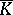ofcomes from an automorphism of;

2)is the splitting field of some family of polynomials with coefficients in(cf. Splitting field of a polynomial);

3) any polynomialwith coefficients in, irreducible overand having a root in, splits ininto linear factors.

For every algebraic extension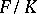there is a maximal intermediate subfieldthat is normal over; this is the field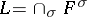, whereranges over all imbeddings ofin. There is also a unique minimal normal extension ofcontaining. This is the composite of all fields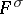. It is called the normal closure of the fieldrelative to. If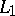andare normal extensions of, then so are the intersection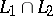and the composite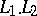. However, whenand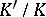are normal extensions,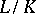need not be normal.

For fields of characteristic zero every normal extension is a Galois extension. In general, a normal extension is a Galois extension if and only if it is separable (cf. Separable extension).

How to Cite This Entry:
Normal extension. Encyclopedia of Mathematics. URL: http://encyclopediaofmath.org/index.php?title=Normal_extension&oldid=15251
This article was adapted from an original article by L.V. Kuz'min (originator), which appeared in Encyclopedia of Mathematics - ISBN 1402006098. See original article